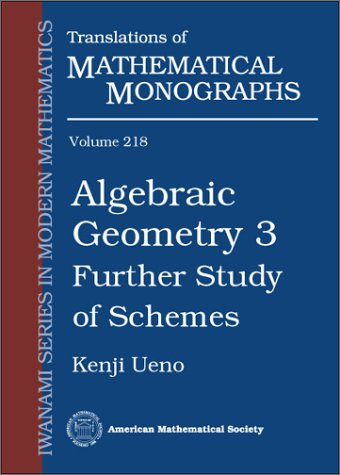## Series Monográficas: Translation of mathematical monographsVol. 223. Noumi, Masatoshi
Painleve equations through symmetry
QA372 N6613

Vol. 247 Marchenko, Vladimir; Slavin, Victor
QA188 M35513

Vol. 222. Magaril-Il’yaev, Georgii G.; Tikhomirov, Vladimir Mikhailovich
Convex analysis : theory and applications
QA639.5 M3413

Vol. 221. Kenmotsu, Katsuei
Surfaces with constant mean curvature
QA644 K4613

Vol. 220. Gelfand, Izrail Moiseevich; Gindikin, Semen Grigorevich; Graev, Mark Iosifovich
Selected topics in integral geometry
QA672 G4513

Vol. 244
Arakelov geometry
QA242.6 M6713

Vol. 243 Saitō, Takeshi
Fermat’s last theorem : basic tools
QA244 S3513

Vol. 218. Ueno, Kenji
Algebraic geometry 3 : further study of schemes
QA564 U35313

Vol. 242 Kurokawa, Nobushige; Kurihara, Masato; Saito, Takeshi
Number theory 3: Iwasawa theory and modular forms
QA241 K37413

Vol. 217. Kashiwara, Masaki

QA614.3 K3713

Vol. 241 Logachev, Oleg A.; Salnikov, Aleksei A.; Yashchenko, V. V.
Boolean functions in coding theory and cryptography
QA268 L6413

Quasipower series and quasianalytic classes of functions
QA295 B3113

Vol. 240 Kato, Kazuya; Kurokawa, Nobushige; Saito, Takeshi
Number theory: Introduction to class field theory
QA241 K37413

Vol. 215. Kimura, Tatsuo
Introduction to prehomogeneous vector spaces
QA186 K5513

Vol. 239 Novikov, Ya; Protasov, V. Yu; Skopina, M.
A wavelet theory
QA403.3 N6813

Vol. 214. Grinblat, Leonid S.
Algebras of sets and combinatorics
QA248 G748

Vol. 238 Vaksman, L. L.
Quantum bounded symmetric domains
QC20.7F84 V3513

Vol. 213. Sachkov, Vladimir Nikolaevich; Tarakanov, Valerii Evgen’evich
Combinatorics of nonnegative matrices
QA188 S31513

Vol. 237. Moriyoshi, Hitoshi; Natsume, Toshikazu
Operator algebras and geometry
QA326 M6613

Vol. 212. Melnikov, A. V.; Volkov, Sergei Nikolaevich; Nechaev, Mikhail Leonidovich

HG4515.3 M4513

Vol. 236. Goldberg, Anatoly A.; Ostrovskii, Iossif V.
Value distribution of meromorphic functions
QA331 G6241

Vol. 211. Ohsawa, Takeo
Analysis of several complex variables
QA331.7 O4713

Vol. 235. Furuta, Mikio
Index Theorem. 1
QA614.92 F8713

Vol. 210. Kohno, Toshitake
Conformal field theory and topology
QC174.52C66 K64

Vol. 234. Chechkin, G. A.; Piatnitski, A. L.; Shamaev, A. S.
Homogenization: methods and applications
QA377 C43913

Vol. 209. Nishiura, Yasumasa
Far-from-equilibrium dynamics
QA852 N5713

Vol. 233. Helemskiæi, A. ëIìA (Aleksandr ëIìAkovlevich)

QA321 H4413

Vol. 208. Matsumoto, Yukio
An introduction to Morse theory
QA331 M39313

Vol. 232. Vasilenko, Oleg Nikolaevich
Number-theoretic algorithms in criptograpgy
QA241 V3713*

Vol. 207. Oshika, Kenichi

QA178 O7413

Vol. 231. Ito, Kiyosi
Essentials of stochastic processes
QA274 I75813

Vol. 206. Shimizu, Yuji

QA564 S5113

Vol. 230. Kono, Akira; Tamaki, Dai
Generalized cohomology
QA612.3 K6513

Vol. 205. Nishikawa, Seiki
Variational problems in geometry
QA614.73 N5713

Vol. 229. Lin'kov, Yu N.
Lectures in mathematical statistics. Parts 1 and 2
QA276.16 L5513

Cohomological analysis of partial differential equations and secondary calculus
QA377 V55

Vol. 228. Zhelobenko, Dmitrii Petrovich
Principal structures and methods of representation theory
QA176 Z5413

Vol. 203. Han, Te Sun

QA268 H35313

Vol. 227. Kawai, Takahiro; Takei, Yoshitsugu
Algebraic analysis of singular perturbation theory
QA372 K37913

Vol. 202. Maslov, Viktor Pavlovich; Omelyanov, Georgi Alexandrovich
Geometric asymptotics for nonlinear PDE. I
QA377 M37513

Hilbert C*-modules
QA326 M3513

Vol. 201. Morita, Shigeyuki
Geometry of differential forms
QA381 M6713

Vol. 200. Prasolov, Viktor Vasilevich
Geometry
QA445 P7313

Vol. 224. Shigekawa, Ichiro

QA274.2 S45513

Vol. 199. Morita, Shigeyuki
Geometry of characteristic classes
QA613.618 M6713

### ¿Quién está en línea?

Hay 226 invitados y ningún miembro en línea

Go to top